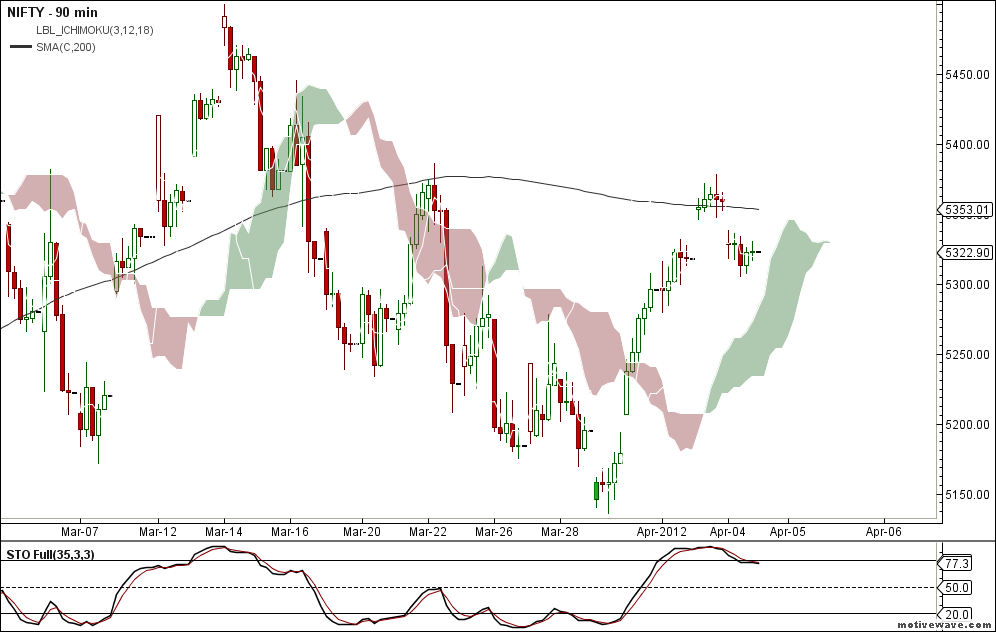# Nifty and Bank Nifty 90 min update for 9th April 2012Nifty and Bank Nifty are still in buy mode trading below the intermediate resistance 200MA line. so as per the last post we had exited our longs and waiting for the next trade. The next trade setup could give a re-entry in long if nifty moves and trades above 200MA line on the 90 min timeframe.

Sell Setup will arrive if both nifty and bank nifty trades below the cloud support zone. Currently cloud supports are near 5242 and 10141. And Interemediate Resistance zone is coming near 5350 and 10440 respectively and re-entry in longs is preferred if nifty and bank nifty breaks intermediate resitance## Nifty and Bank Nifty futures September Overview – III

Nifty and Bank Nifty futures hourly charts are maintaining the buy mode. Currently the supports zone comes around 5799 and 10000 respectively. Reverse to...

## Nifty and Bank Nifty futures September Overview – II

Nifty September futures is in positional buy mode last thursday and currently the support zone comes near 5290. Bank nifty futures turned to positional...

## Nifty and Bank Nifty September Futures overview – I

Nifty september futures turned to positional buy mode and currently the support zone come near 5264 zone. Reverse your position to positional shortly if...

## 2 Replies to “Nifty and Bank Nifty 90 min update for 9th…”

1.jitin says:

Hi raj ,
Looking for a 6 months intraday nse backfill for ami , probably free , anything you have got ?

This is a metastock formula , can it be converted to ami afl?

jitin

Trends:
Click the Trends tab and enter the following formulas in the Bullish and Bearish fields.
Bullish: Mov(C,5,S)>Mov(C,20,S);
Bearish: Mov(C,5,S)<Mov(C,20,S);

Highlights:
Click the Highlights tab, choose New, and enter "3rd Bar" in the Name field. Now change the color in the Color field to Blue. Finally, enter the following formula in the Condition field, and then choose OK.

Symmetry:=.28;
Apex:=(H+L)/2;
WB:=Ref(H,-2)-Ref(L,-2);
Shark:=If((HRef(L,-1) AND Ref(H,-1)Ref(L,-2))=1,If(Apex = (Ref(L,-2)+(WB*Symmetry)) ,1,0),0);
Shark;

Using the same method as above, enter the following 2 highlight formulas.

Name: 2nd Bar

Color: Blue

Condition:
Symmetry:=.28;
Apex:=(H+L)/2;
WB:=Ref(H,-2)-Ref(L,-2);
Shark:=If((HRef(L,-1) AND Ref(H,-1)Ref(L,-2))=1,If(Apex = (Ref(L,-2)+(WB*Symmetry)) ,1,0),0);
Ref(Shark,+1)=1;

Name: 1st Bar

Color: Blue

Condition:
Symmetry:=.28;
Apex:=(H+L)/2;
WB:=Ref(H,-2)-Ref(L,-2);
Shark:=If((HRef(L,-1) AND Ref(H,-1)Ref(L,-2))=1,If(Apex = (Ref(L,-2)+(WB*Symmetry)) ,1,0),0);
Ref(Shark,+2)=1;

Symbols:
Click the Symbols tab, choose New and enter “Shark Buy” in the Name field. Now enter the following formula in the Condition field.

Symmetry:=.28;
Apex:=(H+L)/2;
WB:=Ref(H,-2)-Ref(L,-2);
Shark:=If((HRef(L,-1) AND Ref(H,-1)Ref(L,-2))=1,If(apex = (Ref(L,-2)+(WB*Symmetry)) ,1,0),0);

{Note* The above ValidChk variable makes the Shark signal valid for 25 periods. If the price does not cross above the High value of the base within 25 periods, you will not receive a signal. You can change the number of periods by changing 25 to the number of periods you desire. *}

Click the Graphic tab. Change the symbol in the Graphic field to Buy Arrow. Now change the color in the Color field to Green. Finally, type “Buy” in the Label field, and then choose OK.

Using the Same method as above, enter the following Symbol formula.

Name: Shark Sell

Condition:
Symmetry:=.28;
Apex:=(H+L)/2;
WB:=Ref(H,-2)-Ref(L,-2);
Shark:=If((HRef(L,-1) AND Ref(H,-1)Ref(L,-2))=1,If(apex = (Ref(L,-2)+(WB*Symmetry)) ,1,0),0);
Sellok:=Cross(ValueWhen(1,Shark=1,Ref(L,-2)),C);
Chk:=Cum(Sellok)-ValueWhen(1,Shark=1,Cum(Sellok));

{Note* The above ValidChk variable makes the Shark signal valid for 25 periods. If the price does not cross below the Low value of the base within 25 periods, you will not receive a signal. You can change the number of periods by changing 25 to the number of periods you desire. *}

Sell:= Sellok=1 AND Ref(Chk,-1)=0 AND ValidChk=1;
Sell;

Symbol: Sell Arrow

Color: Red

Label: Sell

After you have finished creating the Symbol formulas, you can attach the Expert to your chart by choosing Expert Advisor from the Tools Menu. Select the Expert called “Shark – 32” from your list of Experts, choose Attach, and then Close.

1.Rajandran R says:

Will try to build that in AFL code format.